# Basic Plane Shapes – Polygons and Circles

### LESSON 2 – POLYGONS

###### TOPIC
• Introduction
• Meaning and Types of Triangles
• Meaning and Types of Quadrilaterals
• Meaning and Types of Polygon
• Meaning and Properties of A Circle
• Lines of Symmetry
• Lesson Evaluation

###### INTRODUCTION

Plane shapes are two dimensional figures such as triangles, quadrilaterals and circles.

Plane shapes are closed flat figure that have different characteristics such as the number of sides angles and corners (vertices).

Plane shapes are two-dimensional, e.g., squares, triangles, and circles. The straight lines that make up a plane shape are called sides. The points where two sides meet are called corners.

A side is a straight line that makes part of the shape, and a corner, or vertex, is where two sides meet.

The basic plane shapes are triangle, square, rectangle, oval, circle, and polygons.

###### TYPES OF PLANE SHAPES

There are different types of plane shapes,

1. Triangles

3. Polygons

4. Circle

###### TRIANGLES

Triangle is a 3 sided plane figure or shape with sum of angles equal to 180°.

###### CHARACTERISTICS (PROPERTIES) OF TRIANGLE

A triangle has following properties,

1. Three sides

2. Three angles

3. Three vertices.

4. The sum of the inside angels equal to 180°.

###### TYPES OF TRIANGLES

The major types of triangles are as follows:

1. Equilateral Triangle

2. Isosceles Triangle

3. Scalene Triangle

1. EQUILATERAL TRIANGLE

An equilateral triangle with all the three sides and angles equal.

Each of the angle is a 60°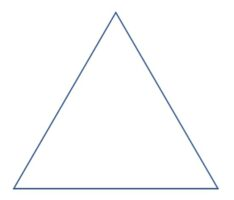2. ISOSCELES TRIANGLE

Isosceles triangle any triangle with two equal sides and two equal angles.

Each of the angle is acute angle, that’s less than 90°.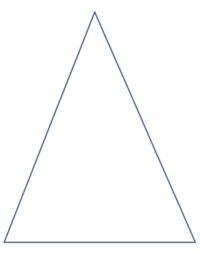3. SCALENE TRIANGLE

A Scalene triangle is any triangle with unequal sides and angles.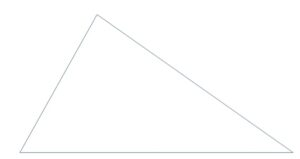There are three types of scalene triangle,

• Right-angled scalene triangle with one of the angle at 90°.
• Acute scalene triangle with all three angles less than 90°.
• Obtuse scalene triangle with one of the angle greater than 90°.

###### CLASS EXERCISE ON TRIANGLES

1. What is a triangle.

2. State 3 types of triangles.

3. Explain the following,

• Equilateral triangle
• Isosceles triangle
• Scalene triangle

4. Draw the 3 types of triangle.

5. Describe the following,

• Right-angled scalene
• Acute scalene triangle
• Obtuse scalene triangle

6. What is the sum of angles in a triangle?

Quadrilateral is a four sided plane figure or shape with sum of angles equal to 360°.

1. Four sides

2. Four angles

3. Four vertices.

4. The sum of the inside angels equal to 360º.

There are different types of quadrilateral are as follows:

1. Square

2. Rectangle

3. Rhombus

4. Parallelogram

5. Trapezium

1. SQUARE

A square is any quadrilateral with four equal sides and and equal angles.

Each of the angle is at 90º.

2. RECTANGLE

A rectangle is any quadrilateral with two opposite sides equal and equal angles.

Each of the angle is at 90º.

3. RHOMBUS

Rhombus is any quadrilateral with four equal parallel sides and equal opposite angles.

Rhombus is not the same with a square.

4. PARALLELOGRAM

A parallelogram is any quadrilateral with equal opposite sides parallel and equal opposite angles.

5. TRAPEZIUM

Trapezium is any quadrilateral with two unequal parallel sides and two non parallel sides.

There are 3 types of trapezium,

• Isosceles trapezium with two equal sides.
• Scalene trapezium with no equal sides.
• Right trapezium with two right angles.

2. State 4 types of quadrilateral?

3. Draw 3 types of quadrilateral?

4. Differentiate between square and rectangle.

5. describe the following:

• Right angle trapezium
• Isosceles trapezium
• Scalene trapezium

7. Rhombus is not a square. Discuss.

8. Each type of quadrilateral is a complete parallelogram except _________.

9. What is the sum of angles in a quadrilateral?

###### POLYGONS

Pentagon is a plane shapes with 3 or more sides and angles.

The following are types of polygons,

1. Triangles

3. Pentagon

4. Hexagon

5. Heptagon

6. Octagon

7. Nonagon

8. Decagon

PENTAGON

Pentagon is a polygon with five equal sides and five equal angles.

Each of the angle is 108° with total of 540°.

HEXAGON

Hexagon is a polygon with six equal sides and six equal angles.

Each of the angle is 120° with total of 720°.

HEPTAGON

Heptagon is a polygon with seven equal sides and seven equal angles.

The sum of the angles is 900°.

Each of the angle is approximately 128.57°.

OCTAGON

Octagon is a polygon with eight equal sides and eight equal angles.

Each of the angle is 135° with total of 1080°.

NONAGON

Octagon is a polygon with nine equal sides and nine equal angles.

Each of the angle is 140° with total of 1260°.

DECAGON

Octagon is a polygon with ten equal sides and ten equal angles.

Each of the angle is 144° with total of 1440°.

###### CLASS EXERCISE ON POLYGONS

1. What is a polygon?

2. State 5 types of polygon you know.

3. Polygon is any shape with 3 or more sides. True or False

4. State all the polygon with 5 or more sides.

5. What is the number of sides and sum of angles in each of the following:

• Triangle
• Pentagon
• Hexagon
• Heptagon
• Nonagon
• Decagon

6. Draw the shape of the following:

• Triangle
• Pentagon
• Hexagon
• Heptagon
• Nonagon
• Decagon

###### CIRCLE

A circle is a round plane shape figure with no corners or edges.

PROPERTIES OF A CIRCLE

The properties of a circle are as follows:

1. Circumstance

3. Diameter

4. Chord

5. Arc

6. Sector

7. Secant

8. Tangent

9. Semicircle

###### PROPERTIES OF A CIRCLE

1. CIRCUMFERENCE

A circle is the distance or line round a circle.

Radius is the line from the centre point of a circle to the circumstance.

It is half of the diameter.

3. DIAMETER

Diameter is the line that passes through the centre point of a circle and touches the two points on the circumference.

4. CHORD

A chord is a straight line that joins any two points on the circumference.

5. ARC

An arc is a part of the circumference of a circle

There are two types of arc,

• Major arc
• Minor arc

Major arc is the larger of the circumference while the minor is  of the smaller part of the circumference.

6. SECTOR

A sector is a part of a circle enclosed with two radii that connects the arc of a circle.

7. SECANT

Secant is a line that cuts the circle into two parts.

Each part is not equal to the other.

8. TANGENT

A tangent is the line that touches the circle of a circle.

9. SEMICIRCLE

Semicircle is the half of the circle.

###### CLASS EXERCISE ON CIRCLE

1. What is a circle?

2. State 5 properties of a circle.

3. differentiate between a radius and a diameter.

4. Explain the following:

• Circumference
• Diameter
• Arc
• Sector
• Chord
• Secant
• Tangent

5. Draw and indicate 5 properties of a circle.

###### LINES OF SYMMETRY

ACTIVITY 1 – INTRODUCTION

A line of symmetry is a line that a shape exactly into two.

For example,

If you fold a shape such a square into two and both parts would match exactly, the line in between is known line of symmetry.

ACTIVITY 2 – FOLDING SHAPES WITH 3 SIDES OR MORE

Teacher organizes pupils in groups or pairs depending on the size of the class to determine the lines of symmetry by folding or cutting.

1. Triangles

There are 3 major types of triangles,

Equilateral triangle has 3 lines of symmetry.

Isosceles triangle has one line of symmetry.

Scalene Triangle has zero line of symmetry.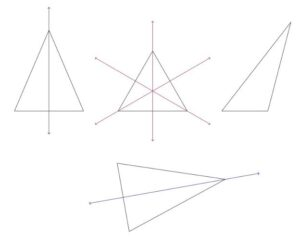There are different types of quadrilateral are as follows:

1. Square has 4 lines of symmetry.

2. Rectangle has 2 lines of symmetry.

3. Rhombus has 2 lines of symmetry.

4. Parallelogram has no line of symmetry.

5. Trapezium has no line of symmetry.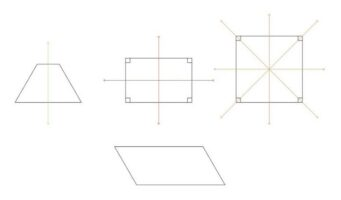3. Pentagon

Polygon has 5 lines of symmetry.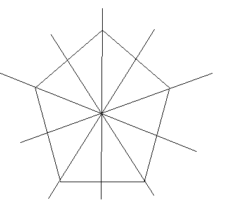4. Hexagon

Hexagon has 6 Lines of symmetry.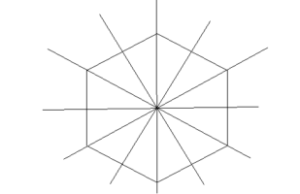5. Heptagon

Heptagon has 7 lines of symmetry.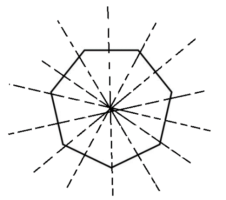6. Octagon

Octagon has 8 lines of symmetry.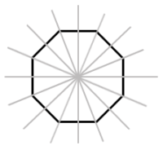7. Nonagon

Nonagon has 9 lines of symmetry.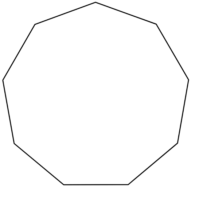8. Decagon

Decagon has 10 lines of symmetry.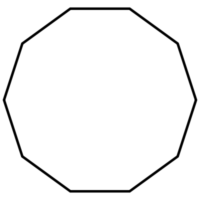###### LESSON EVALUATION

1. explain the meaning of plane shape with examples.

2. state 5 types of plane shapes.

3. what is a triangle?

4. list and explain each types of triangles.

6. state and explain each types of quadrilateral.

7. what is a polygon?

8. write short note on the following:

• pentagon
• hexagon
• heptagon
• nonagon
• decagon

9. state the number of lines of symmetry for each of the following:

• triangle